# 基于VLBM方法研究柴油机多孔微粒捕集器渗流机理Study on Seepage Mechanism of Diesel Porous Particulate Filter Based on VLBM

DOI: 10.12677/APF.2018.84008, PDF, HTML, XML, 下载: 418  浏览: 951  国家自然科学基金支持

Abstract: Diesel engines have been widely used in vehicles, ships and other power plants since they were invented because of their good power and economic advantages. At the same time, diesel particu-late emissions also bring serious pollution problems, causing widespread concern. In this paper, the influence of porous media filter structure and inlet velocity in the flow field of the trap passage model is discussed on the pore scale by using the volumetric lattice Boltzmann method. It provides a new idea for numerical simulation of diesel particulate trap. The results show that the larger the porosity is, the more stable the flow field is and the smaller the pressure difference between inlet and outlet is. Compared with porosity, the thickness of the filter has less influence on the flow field. However, the greater the thickness of the filter, the greater the pressure difference between the inlet and outlet channels is. The larger the inlet velocity is, the more turbulent the flow field is, the greater the pressure difference is.

1. 前言

2. 物理模型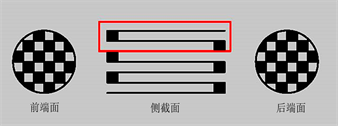Figure 1. Wall flow particulate trap structure

1. 壁流式微粒捕集器结构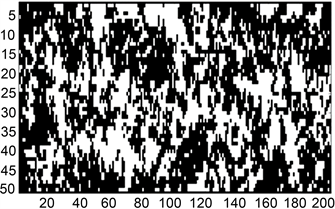Figure 2. Two dimensional model of porosity 0.4

2. 孔隙率0.4的二维模型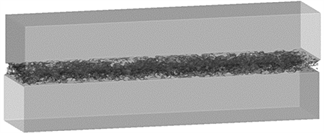Figure 3. There dimensional model of porosity 0.4

3. 孔隙率0.4的三维模型

3. 模拟方法

3.1. 方法概述

Yu等人近年来提出了体积格子玻尔兹曼方法(VLBM)，并通过验证证实VLBM方法对于解决如多孔介质、移动边界等复杂的结构模型具有一定的优势。通过引入一个定义为固体基质部分占单元格体积比例的体积参数 $P\left(x,t\right)$ 来描述多孔介质中基质与孔隙部分，并将多孔介质中单元格分成三类：1) 固体单元格 $\left(P\left(x,t\right)=1\right)$ ；2) 流体单元格 $\left(P\left(x,t\right)=0\right)$ ；3) 边界单元格点 $\left(0 ，如图4所示。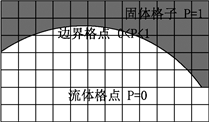Figure 4. Cell classification

4. 单元格分类

3.2. 方法介绍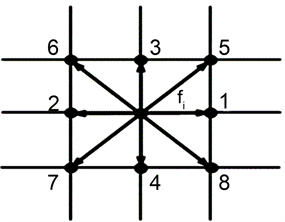(a)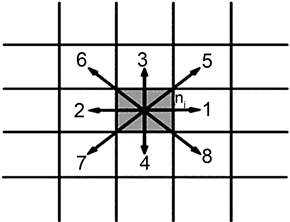(b)

Figure 5. (a) Traditional LBM lattice model; (b) VLBM lattice model

5. (a) 传统LBM格子模型；(b) VLBM格子模型

${n}_{i}\left(x,t\right)={f}_{i}\left(x,t\right)×\left[1-\text{P}\left(x,t\right)\right]×V$ (1)

${n}_{i}\left(x+{e}_{i}\Delta t,t+\Delta t\right)={n}_{i}\left(x,t\right)+{\Omega }_{i}\left(x,t\right)$ (2)

${\Omega }_{i}\left(x,t\right)=-1/\tau \left[{n}_{i}\left(x,t\right)-{n}_{i}^{eq}\left(x,t\right)\right]$ (3)

${n}_{i}^{eq}\left(x,t\right)=N\left(x,t\right){\omega }_{i}\left[1+\frac{{e}_{i}\cdot u}{{c}_{s}^{2}}+\frac{{\left({e}_{i}\cdot u\right)}^{2}}{2{c}_{s}^{4}}+\frac{{\left(u\right)}^{2}}{2{c}_{s}^{2}}\right]$ (4)

${{n}^{\prime }}_{i}\left(x,t\right)={n}_{i}\left(x,t\right)+{\Omega }_{i}\left(x,t\right)$ (5)

${{n}^{″}}_{i}\left(x,t+\Delta t\right)=\left[1-P\left(x,t\right)\right]{{n}^{\prime }}_{i}\left(x-{e}_{i}\Delta t,t\right)+P\left(x-{e}_{{}_{i*}}\Delta t,t\right){{n}^{\prime }}_{i*}\left({x}_{i},t\right)$ (6)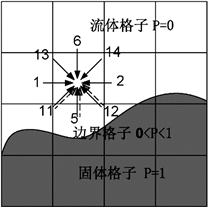Figure 6. Sketch map of streaming steps

6. 迁移步示意图

$\rho \left(x,t\right){=}^{\text{​}}\sum {n}_{i}\left(x,t\right)/\left[1-P\left(x,t\right)\right]$ (7)

$u\left(x,t\right)=\sum {e}_{i}{n}_{i}\left(x,t\right)/\sum {n}_{i}\left(x,t\right)$ (8)

$p\left(x,t\right)-{p}_{0}={c}_{s}^{2}\left[\rho \left(x,t\right)\right]-{\rho }_{0}$ (9)

4. 结果讨论和分析

4.1. 过滤体孔隙度对流场的影响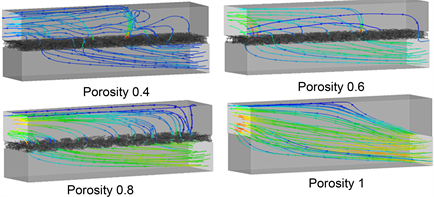Figure 7. Streamline of channel under different porosity

7. 不同孔隙度下孔道的流线图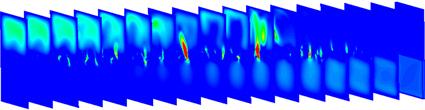Figure 8. Flow fields in X direction at 0.4 porosity

8. 孔隙度为0.4时X方向各截面流场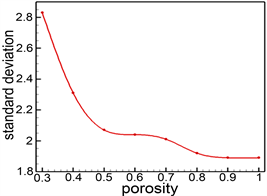(a)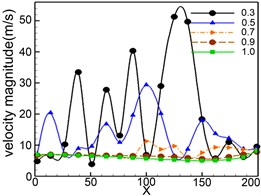(b)

Figure 9. (a) Standard deviation of different porosity; (b) Maximum velocity of cross section in X direction with different porosity

9. (a) 不同孔隙度标准差；(b) 不同孔隙度X方向截面最大流速Figure 10. Pressure difference between inlet and outlet under different porosity

10. 不同孔隙度下进出口孔道压差

4.2. 过滤体厚度对流场的影响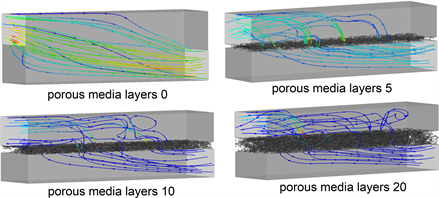Figure 11. Streamlines of different thickness at porosity 0.4

11. 孔隙度0.4时孔道在不同厚度流线图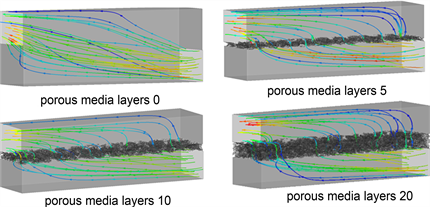Figure 12. Streamlines of different thickness at porosity 0.8

12. 孔隙度0.8时孔道在不同厚度流线图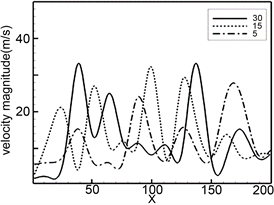(a)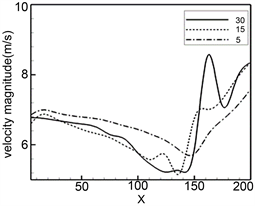(b)

Figure 13. (a) The maximum velocity of cross section in X direction at porosity 0.4; (b) the maximum velocity of cross section in X direction at porosity 0.8

13. (a) 孔隙度为0.4时X方向截面最大流速；(b) 孔隙度为0.8时X方向截面最大流速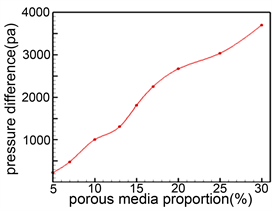(a)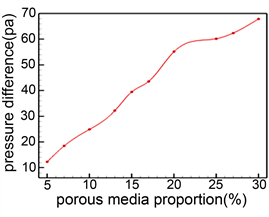(b)

Figure 14. (a) Pressure difference between inlet and outlet at porosity 0.4; (b) pressure difference between inlet and outlet at porosity 0.8

14. (a) 孔隙度为0.4时进出口孔道压差；(b) 孔隙度为0.8时进出口孔道压差

30层的过程中，进出口孔道压差从200多pa一直上升到3000多pa。当孔隙度为0.8时，随着过滤体厚度的增加，压差也随之上升。由于孔隙度较大，与孔隙度为0.4的情况相比，进出口孔道之间的压差要小很多，即使当厚度达到30层，压差也仅仅只有60多pa。综上所述，多孔介质过滤体厚度大小对微粒捕集器流线形状影响较小，但是随着过滤体厚度的增加，进出口孔道压差随之上升，因此在微粒捕集器结构的设计优化中，也应该考虑到过滤体厚度。

4.3. 进口速度对于流场的影响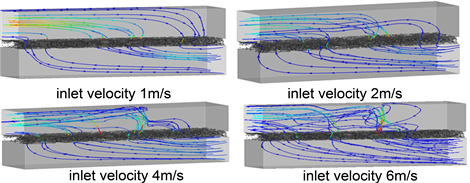Figure 15. Streamline under different inlet speeds

15. 不同进口速度下孔道流线图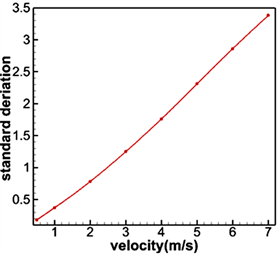Figure 16. Standard deviation at different speeds

16. 不同速度下的标准差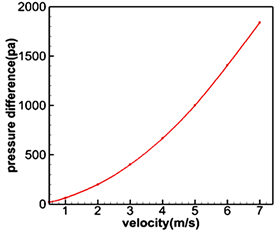Figure 17. Pressure difference under different inlet speeds

17. 不同进口速度下孔道间压差

5. 总结与展望

NOTES

*通讯作者。

  王逢瑚, 等. 柴油车尾气微粒捕集器技术研究现状及发展趋势[J]. 小型内燃机与车辆技术, 2010, 39(1): 92-96.  资新运, 等. 柴油机微粒捕集器技术发展现状[J]. 环境科学与技术, 2011(S2): 143-147.  甘星星. 浅谈汽车尾气排放问题及节能减排的方法[J]. 内燃机与配件, 2018(1): 254-255.  刘恒语. 壁流式微粒捕集器连续再生特性研究[D]. 湖南大学, 2012.  Konstandopoulos, A.G., et al. (2007) Advances in the Science and Technology of Diesel Particulate Filter Simulation, In: Automotive Emission Control, 213-294.  聂存庆. 柴油机微粒捕集器关键技术研究[J]. 科技与创新, 2014(3): 6-7.  付小丹, 发动机碳烟颗粒捕集器的性能研究[D]. 广东工业大学, 2007.  Yu, H., et al. (2014) Mass-Conserved Volumetric Lattice Boltzmann Method for Complex Flows with Willfully Moving Boundaries. Physical Review E Statistical Nonlinear & Soft Matter Physics, 89, 063304.  An, S., Yu, H. and Yao, J. (2017) GPU-Accelerated Volumetric Lattice Boltzmann Method for Porous Media Flow. Journal of Petroleum Science and Engineering, 156, 546-552.  伏军. 微粒捕集器喷油助燃再生过程热工参数建模及控制系统研究[D]. 湖南大学, 2011.  Qian, Y.H., D’Humières, D. and Lallemand, P. (2007) Lattice BGK Models for Navier-Stokes Equation. Europhysics Letters (Epl), 17, 479.  姜大海, 等. 柴油机颗粒捕集器压力损失的研究[J]. 汽车工程, 2013, 35(5): 397-402.  王鹏伟. 多孔介质微流动特性的实验研究[D]. 中国计量学院, 2014.